1系统概述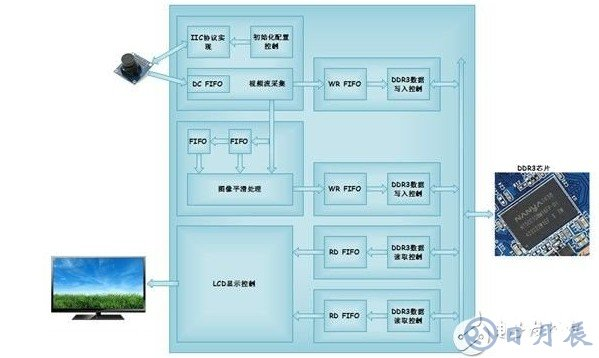2图像平滑与滤波

2.1 基本概念

2.2 滤波算法

•限幅滤波法（又称程序判断滤波法）

• 中位值滤波法

• 算术平均滤波法

• 高斯滤波法

• 递推平均滤波法（又称滑动平均滤波法）

• 中位值平均滤波法（又称防脉冲干扰平均滤波法）

• 限幅平均滤波法

• 一阶滞后滤波法

• 加权递推平均滤波法

• 消抖滤波法

• 限幅消抖滤波法

•卡尔曼滤波（非扩展卡尔曼）

2.3 均值滤波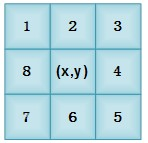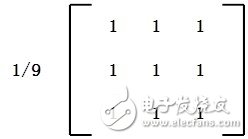2.4 加权均值滤波器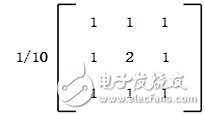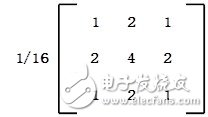clear

clc

I=im2double(I1);

[m,n,c]=size(I);

A=zeros(m,n,c);

%           1   2   1

%   1/16 *  2   4   2

%           1   2   1

%for R

for i=2:m-1

for j=2:n-1

A(i,j,1)=I(i-1,j-1,1)+I(i+1,j-1,1)+I(i-1,j+1,1)+I(i+1,j+1,1)+2*I(i+1,j,1)+2*I(i-1,j,1)+2*I(i,j+1,1)+2*I(i,j-1,1)+4*I(i,j,1);

end

end

%for G

for i=2:m-1

for j=2:n-1

A(i,j,2)=I(i-1,j-1,2)+I(i+1,j-1,2)+I(i-1,j+1,2)+I(i+1,j+1,2)+2*I(i+1,j,2)+2*I(i-1,j,2)+2*I(i,j+1,2)+2*I(i,j-1,2)+4*I(i,j,2);

end

end

%for B

for i=2:m-1

for j=2:n-1

A(i,j,3)=I(i-1,j-1,3)+I(i+1,j-1,3)+I(i-1,j+1,3)+I(i+1,j+1,3)+2*I(i+1,j,3)+2*I(i-1,j,3)+2*I(i,j+1,3)+2*I(i,j-1,3)+4*I(i,j,3);

end

end

B=A/16;

%output

imwrite(B,'lena.tif','TIf');

imshow('.\lena.jpg');TItle('origin image');figure

imshow('lena.TIf');TItle('image after average filter')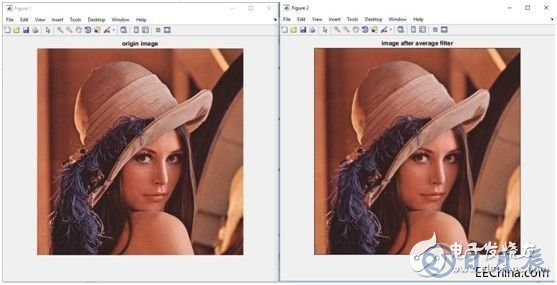Matlab源码、Lena.jpg原图和比对图存放在at7_img_ex01\matlab文件夹下。

• ·上一篇：如何利用51单片机控制液晶显示
·下一篇：C51单片机串口驱动设计的常见问题解析
• 其他最新资讯
深圳市日月辰科技有限公司
地址：深圳市宝安区松岗镇潭头第二工业城A区27栋3楼
电话：0755-2955 6626
传真：0755-2978 1585
手机：131 1300 0010